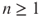### GRAPH-THEORETIC MODELS I

In Chapter 20, we used the Q-matrix to extract some interesting properties of both Fibonacci and Lucas numbers. We will now employ it to construct graph-theoretic models for them, and then explore some of the well-known identities. These models enable us to study Fibonacci and Lucas numbers in a different perspective.

To begin with, recall thatwhere.

We are now ready for the models.

## 21.1 A GRAPH-THEORETIC MODEL FOR FIBONACCI NUMBERS

The Q-matrix can geometrically be translated into a connected graph G with two verticesand; see Figure 21.1. In fact, Q is the adjacency matrixof the graph, wheredenotes the number of edges fromto , and .

Get Fibonacci and Lucas Numbers with Applications, Volume 1, 2nd Edition now with the O’Reilly learning platform.

O’Reilly members experience books, live events, courses curated by job role, and more from O’Reilly and nearly 200 top publishers.